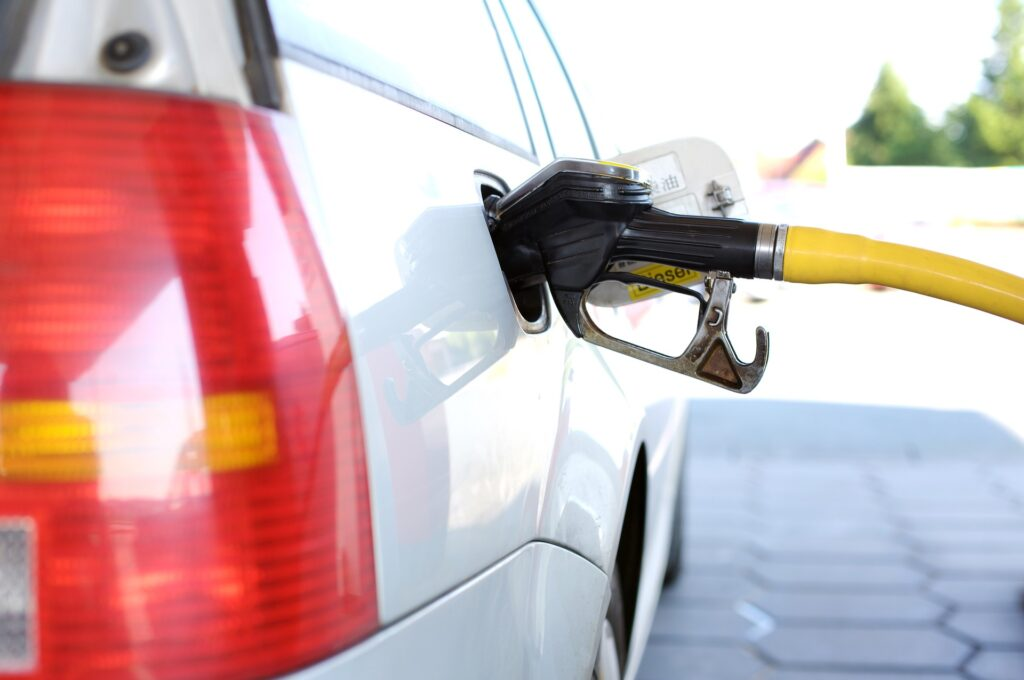## Discussion: Comparing Hydrocarbon Efficiency

Enthalpy is used to evaluate the energy contained within chemical bonds and substances. Combustion reactions are especially useful in these analyses, because humans use them to generate so much of our daily energy. The combustion of these fuels generally follows the pattern of the chemical reaction shown here:

“hydrocarbon fuel” + __$\text{O}_{2}$(g) $\rightarrow$  __$\text{CO}_{2}$(g) + __$\text{H}_{2}\text{O}$(g)For this activity, we want to compare the efficiency of different fuel sources. Pick one of these common hydrocarbons used for fuel and do a little research about it:

• Methane
• Ethane
• Propane
• Butane
• Pentane
• Octane (part of gasoline)
• Dodecane (part of gasoline)
• Eicosane (part of jet fuel)

Based on the fuel you have selected, complete the following steps:

1. Write a correctly balanced chemical equation for the reaction for enthalpy of formation
2. Calculate the enthalpy of the combustion reaction from enthalpy of formation values
3. Calculate the heat liberated by burning 100.0g of the fuel
4. Post your findings to the discussion board
5. Read through others’ posts and compare the efficiency of your fuel to theirs
6. Make some comparisons and write one more post with your thoughts on the similarities and differences you noticed between fuels